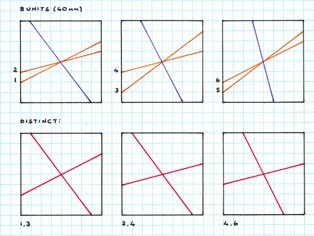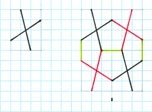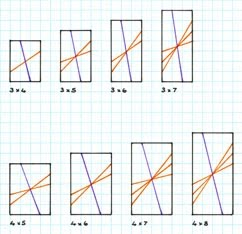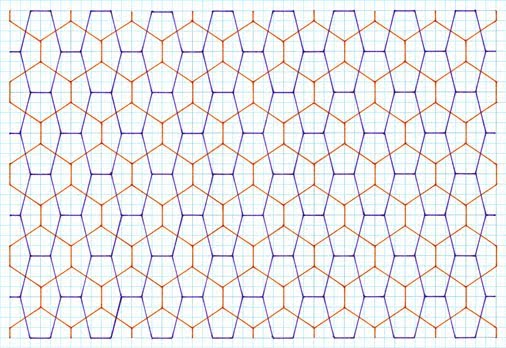Cairo Tiling‎ > ‎

### Study 2

 PremiseThis continues with the ‘reflected stick cross’ premise as in Study 1, but here, rather than a symmetrical cross of equal arms in a square matrix as according to the intersections of the unit grid, I instead consider an asymmetric cross. As can be seen, this divides the square matrix into four different regions, two of which are distinct, and so consequently when the square matrix is reflected it thus gives two different pentagons. As can be seen, the pentagons are symmetric, of 2, 2, 1 side lengths for any given tiling. Of note is that the angles are no longer 90°. The pentagons range from being two of very much alike to widely different ones, with decidedly short and long bases, of which incidentally can this be described simply as of short or long bases. Examples of 5-10 unit squares are shown, a five unit square being the minimum, of which this as this is another example of an ‘infinite’ type, of which I cease at 10 units, with 20 distinct examples, this being more than sufficient to see the ‘nature’ of the eventual tiling.Figure 1: PremiseFigure 2: 5 x 5Figure 3: 6 x 6Figure 4: 7 x 7Figure 5: 8 x 8Figure 6: 9 x 9Figure 7: 10 x 10Figure 8: Set of 20TilingsUpon having shown the premise of this particular creation, I here show these as actual tilings thereof. Note that for the sake of conciseness, I show just the first few examples, as there is an infinite number. Exactly, I show five examples, which more than suffices as an indication as to their nature.Figure 9:Figure 10:Figure 11:Figure 12: Figure 13: CommentsAs can be seen, this particular creation process gives two different pentagons, both symmetrical. Of interest is that each pentagon has three different side lengths, of 2, 2, 1 for any given example. Of note is that the angles are no longer 90°, as occurred with the preceding ‘symmetric cross’ of ‘Study 1’.The pentagons range from being two of very much alike to widely different ones, with decidedly short and long bases (the ‘1’ side above), of which incidentally can this be described simply as of short or long bases. Aesthetically, these ‘extreme’ examples are lacking, in that these are furthest away from an ‘average’ pentagon. The subsidiary hexagons are at right angles (which as I later show with other, different premises is not always so) which again is aesthetically pleasing.    As can be seen, as a type, with the increase in number of pentagons, the ‘two pentagon tilings’ lack the elegance of a single one. Certainly, they are interesting, but aesthetically pale in comparison.A variation of the above is with a rectangular format, below:PremiseThis continues with the ‘reflected stick cross’ premise as in Study 1, but with a different matrix, namely that of a rectangle, as according to the intersections of the unit grid. Of necessity, the resulting stick cross is asymmetric. As can be seen, this divides the rectangular matrix into four different regions, two of which are distinct, and so consequently when the rectangular matrix is reflected it thus gives two different pentagons. As can be seen, the pentagons are symmetric, of three different side lengths, of 2, 2, 1 proportions for any given tiling. Of note is that the angles are no longer 90° as with Study 1.Figure 14: PremiseFigure 15: 3 x 4 to 3 x 7; 4 x 5 to 4 x 8Figure 16: 3 x 4 to 3 x 7; 4 x 5 to 4 x 8Figure 17: 4 x 5 to 4 x 8TilingsAs above I am here more circumspect in the number of tilings I show – just three. The reason for this is that I consider this particular premise, of rectangle grids, as lacking in aesthetics (see Comments), of which I see no need to show these to the same depth as with preceding studies, of 18 examples. Broadly, a few suffice to show the general principle, and so as they are labour intensive to draw, for reasons of both time saving and their lack of aesthetic appeal, further examples are not shown.Figure 18:Figure 19:Figure 20:CommentsAs can be seen, this particular creation process gives two different pentagons, both symmetrical. Of interest is that each pentagon has three different side lengths, of 2, 2, 1 proportions for any given example. Of note is that the angles are no longer 90°, as occurred with the preceding Study 1 - ‘symmetric cross and square matrix’. The pentagons range from being two of broadly alike to widely different ones, with decidedly short and long bases (the ‘1’ side above), of which incidentally can this be described simply as of short or long bases. Aesthetically, these ‘extreme’ examples are lacking, in that these are furthest away from an ‘average’ pentagon. The subsidiary hexagons are at right angles (which as I later show with other, different premises is not always so) which again is aesthetically pleasing.    Typically, due to the nature of the (rectangular) matrix, with increasing unit size of the matrix; 3 x 4, 3 x 5, 3 x 6… this thus results in long, narrow pentagons, the aesthetics of which are lacking (of which I discuss below). This being so, I did not pursue further examples, although I do show these in a theoretical sense, with the construction premise.    As can be seen, as a type, with the increase in number of pentagons, the ‘two pentagon tilings’ lack the elegance of a single one. Furthermore, due to the rectangular matrix, the pentagons are typically ‘elongated’, and so lack appeal. In effect, these can be regarded as ‘stretched’ squares, and so of limited interest aesthetically. Certainly, they are, loosely, ‘interesting’, but aesthetically pale in comparison with other Cairo tilings, even within the ‘two pentagon’ category.    Another aspect to this is that although of a different construction to the preceding Study 2 (two pentagon examples) i.e. square, as against rectangle, the same outcome as to the ‘type’ of tiling occurs, i.e. 2 pentagons, both symmetrical, same proportions of sides (2, 2, 1), so although composed differently, they are of the same ‘type’.    In essence, this study is lacking in aesthetics (at least in comparison to the related procedures of Study 1. Firstly, it is composed of two pentagons, whereas one is aesthetically better. Secondly, the matrix is no longer of the most basic type of quadrilateral, a square, but of a rectangle.Created 12 September 2011